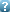Landau Days 2016 Landau Days 2016 June, 20-22 Chernogolovka, RussiaCompact Equations for Water waves: temporal and spatial Date/Time: 12:10 21-Jun-2016 Abstract: We derive very simple compact equations for unidirectional gravity water waves. For such waves, as it is well-known, the coefficient of nontrivial four-wave interaction is identically zero. This fact allows essentially to simplify the Zakharov equation applying a canonical transformation. Obviously this transformation is not unique. We suggest a specific form of such transformation that allows to derive a remarkably simple form of the Zakharov equation. One can name it as the super compact equation. This equation is very traightforward and includes nonlinear wave term (\`{a} la NLSE) and advection term (may describe pre-breaking wave). Moreover, this equation allows also to derive spatial version of water waves equation which can be used to describe experiments it the flume. Authors Dyachenko Alexander I.(Presenter) (no additional information) Zakharov Vladimir E.(no additional information) Kachulin Dmitry Igorevich(no additional information) © 2012, Landau Institute for Theoretical Physics RAS www.itp.ac.ru Contact webmaster